# 性阿就感的Promise，拥抱ta然后扒光ta## 1. How Promise?

• 创建Promise

``````let p = new Promise
console.log(p) // TypeError: Promise resolver undefined is not a function
``````
• 参数

``````let p =new Promise(function(resolve, reject){
if(/* 异步操作成功 */){
resolve(data)
}else{
reject(err)
}
})
``````
• state

• resolve/reject 方法

resolve方法可以将pending转为fulfilled，reject方法可以将pending转为rejected。

• then方法

``````p.then(function(data){ // resolve方法会将参数传进成功的回调
console.log(data)
}, function(err){      // reject方法会将失败的信息传进失败的回调
console.log(err)
})
``````举个栗子
``````let p = new Promise(function(resolve, reject){
setTimeout(function(){
let num = Math.random()
if (num > 0.5) {
resolve(num)
}else{
reject(num)
}
}, 1000)
})
p.then(function(num){
console.log('大于0.5的数字：', num)
},function(num){
console.log('小于等于0.5的数字', num)
})
// 运行第一次：小于等于0.5的数字 0.166162996031475
// 运行第二次：大于0.5的数字： 0.6591451548308984
...
``````

• 链式调用

``````p.then(function(num){
return num
},function(num){
return num
}).then(function(num){
console.log('大于0.5的数字：', num)
},function(num){
console.log('小于等于0.5的数字', num)
})
``````
• catch方法

catch方法等同于.then(null, reject)，可以直接指定失败的回调（支持接收上一个then发生的错误）

• Promise.all()

Promise.all能将多个Promise实例包装成一个Promise实例

``````let Promise1 = new Promise(function(resolve, reject){})
let Promise2 = new Promise(function(resolve, reject){})
let Promise3 = new Promise(function(resolve, reject){})

let p = Promise.all([Promise1, Promise2, Promise3])

p.then(funciton(){
// 三个都成功则成功
}, function(){
// 只要有失败，则失败
})
``````

1、当Promise1, Promise2, Promise3的状态都为成功态，则p为成功态； 2、当Promise1, Promise2, Promise3中有任意一个为失败态，则p为失败态；

• Promise.race()

• Promise.resolve()

Promise.resolve('成功')等同于new Promise(function(resolve){resolve('成功')})

• Promise.reject()

Promise.reject('出错了')等同于new Promise((resolve, reject) => reject('出错了'))

## 2. Why Promise?

``````\$.get('url', {data: data}, function(result){
console.log('成功', result)// 成功的回调，result为异步拿到的数据
});
``````

``````\$.get('url', {data: data}, function(result1){
\$.get('url', {data: result1}, function(result2){
\$.get('url', {data: result2}, function(result3){
\$.get('url', {data: result3}, function(result4){
......
\$.get('url', {data: resultn}, function(resultn+1){
console.log('成功')
}
}
}
}
});
``````

1.txt的内容：

``````2.txt
``````

2.txt的内容：

``````3.txt
``````

3.txt的内容：

``````完成
``````

js代码：

``````let readFile = require('fs').readFile; // 加载node内置模块fs 利用readFile方法异步访问文件
function getFile(url){  // 创建一个读取文件方法
return new Promise(function(resolve, reject){  // 返回一个Promise对象
resolve(data)  // 调用成功的方法
})
})
}
getFile('1.txt').then(function(data){  // then方法进行链式调用
console.log(data)  // 2.txt
return getFile(data)    //拿到了第一次的内容用来请求第二次
}).then(function(data){
console.log(data)  // 3.txt
return getFile(data)  //拿到了第二次的内容用来请求第三次
}).then(function(data){
console.log(data)  // 完成
})
``````

（这里我们先不必搞懂代码，下面会介绍具体用法）

## 3. What Promise?

• 实现resolve、reject方法，then方法和状态机制

``````// myPromise
function Promise(executor){ //executor是一个执行器（函数）
let _this = this // 先缓存this以免后面指针混乱
_this.status = 'pending' // 默认状态为等待态
_this.value = undefined // 成功时要传递给成功回调的数据，默认undefined
_this.reason = undefined // 失败时要传递给失败回调的原因，默认undefined
function resolve(value) { // 内置一个resolve方法，接收成功状态数据
// 上面说了，只有pending可以转为其他状态，所以这里要判断一下
if (_this.status === 'pending') {
_this.status = 'resolved' // 当调用resolve时要将状态改为成功态
_this.value = value // 保存成功时传进来的数据
}
}
function reject(reason) { // 内置一个reject方法，失败状态时接收原因
if (_this.status === 'pending') { // 和resolve同理
_this.status = 'rejected' // 转为失败态
_this.reason = reason // 保存失败原因
}
}
executor(resolve, reject) // 执行执行器函数，并将两个方法传入
}
// then方法接收两个参数，分别是成功和失败的回调，这里我们命名为onFulfilled和onRjected
Promise.prototype.then = function(onFulfilled, onRjected){
let _this = this;   // 依然缓存this
if(_this.status === 'resolved'){  // 判断当前Promise的状态
onFulfilled(_this.value)  // 如果是成功态，当然是要执行用户传递的成功回调，并把数据传进去
}
if(_this.status === 'rejected'){ // 同理
onRjected(_this.reason)
}
}
module.exports = Promise  // 导出模块，否则别的文件没法使用
``````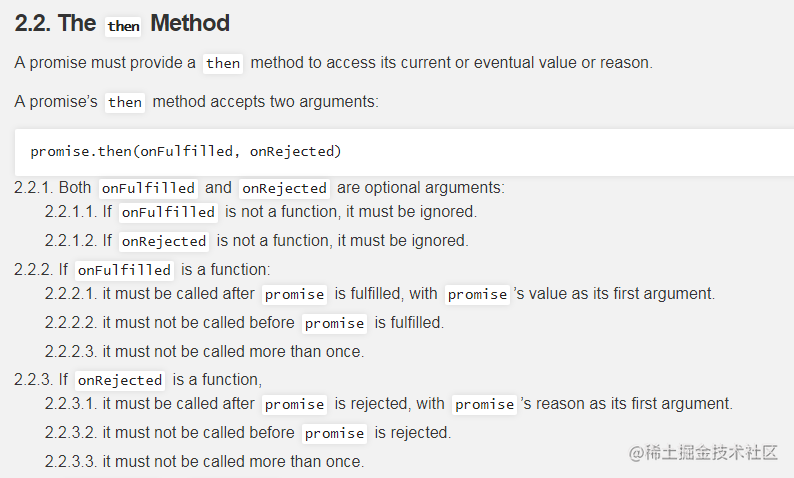``````let Promise = require('./myPromise')  // 引入模块
let p = new Promise(function(resolve, reject){
resolve('test')
})
p.then(function(data){
console.log('成功', data)
},function(err){
console.log('失败', err)
})
// 成功 test
``````

``````let Promise = require('./myPromise')  // 引入模块
let p = new Promise(function(resolve, reject){
reject('test')
})
p.then(function(data){
console.log('成功', data)
},function(err){
console.log('失败', err)
})
// 失败 test
``````

``````let p = new Promise(function(resolve, reject){
setTimeout(function(){
resolve(100)
}, 1000)
})
p.then(function(data){
console.log('成功', data)
},function(err){
console.log('失败', err)
})
// 不会输出任何代码
``````

• 实现异步

``````_this.onResolvedCallbacks = []; // 存放then成功的回调
_this.onRejectedCallbacks = []; // 存放then失败的回调
``````

then方法加一个pending时的判断

``````if(_this.status === 'pending'){
// 每一次then时，如果是等待态，就把回调函数push进数组中，什么时候改变状态什么时候再执行
_this.onResolvedCallbacks.push(function(){ // 这里用一个函数包起来，是为了后面加入新的逻辑进去
onFulfilled(_this.value)
})
_this.onRejectedCallbacks.push(function(){ // 同理
onRjected(_this.reason)
})
}
``````

``````function resolve(value) {
if (_this.status === 'pending') {
_this.status = 'resolved'
_this.value = value
_this.onResolvedCallbacks.forEach(function(fn){ // 当成功的函数被调用时，之前缓存的回调函数会被一一调用
fn()
})
}
}
function reject(reason) {
if (_this.status === 'pending') {
_this.status = 'rejected'
_this.reason = reason
_this.onRejectedCallbacks.forEach(function(fn){// 当失败的函数被调用时，之前缓存的回调函数会被一一调用
fn()
})
}
}
``````

• 处理错误

``````new Promise(function(resolve, reject){
throw new Error('错误')
}).then(function(){

},function(err){
console.log('错误:', err)
})
// 错误: Error: 错误
``````

``````try{
executor(resolve, reject)
}catch(e){ // 如果捕获发生异常，直接调失败，并把参数穿进去
reject(e)
}
``````
• 实现then的链式调用（难点）

``````let p1 = new Promise(function(resolve, reject){
resolve()
})
let p2 = p1.then(function(data){ //这是p1的成功回调，此时p1是成功态
throw new Error('错误') // 如果这里抛出错误，p2应是失败态
})
p2.then(function(){

},function(err){
console.log(err)
})
// Error: 错误
``````

``````// 改动then
let promise2;
if (_this.status === 'resolved') {
promise2 = new Promise(function (resolve, reject) {
// 可以凑合用，但是是有很多问题的
try {
let x = onFulfilled(_this.value)
resolve(x)
} catch (e) {
reject(e)
}
})
}
if (_this.status === 'rejected') {
promise2 = new Promise(function (resolve, reject) {
// 可以凑合用，但是是有很多问题的
try {
let x = onRjected(_this.reason)
resolve(x)
} catch (e) {
reject(e)
}
})
}
if(_this.status === 'pending'){
promise2 = new Promise(function (resolve, rejec
_this.onResolvedCallbacks.push(function(){
// 可以凑合用，但是是有很多问题的
try {
let x = onFulfilled(_this.value)
resolve(x)
} catch (e) {
reject(e)
}
})
_this.onRejectedCallbacks.push(function(){
// 可以凑合用，但是是有很多问题的
try {
let x = onRjected(_this.reason)
resolve(x)
} catch (e) {
reject(e)
}
})
})
}
return promise2
``````

``````let p = new Promise(function(resolve, reject){
resolve(data)
})
p.then(function(data){
return xxx // 这里返回一个值
}, function(){

}).then(function(data){
console.log // 这里会接收到xxx
}, function(){

})
// 以上代码中第一次then的返回值就是源码内第一次调用onRjected的返回值，可以用一个x来接收
``````

1、前一次then返回一个普通值，字符串数组对象这些东西，都没问题，只需传给下一个then，刚才的方法就够用。

2、前一次then返回的是一个Promise，是正常的操作，也是Promise提供的语法糖，我们要想办法判断到底返回的是啥。

3、前一次then返回的是一个Promise，其中有异步操作，也是理所当然的，那我们就要等待他的状态改变，再进行下面的处理。

4、前一次then返回的是自己本身这个Promise

``````var p1 = p.then(function(){// 这里得用var，let由于作用域的原因会报错undefined
return p1
})
``````

5、前一次then返回的是一个别人自己随便写的Promise，这个Promise可能是个有then的普通对象，比如{then:'哈哈哈'}，也有可能在then里故意抛错（这种蛋疼的操作我们也要考虑进去）。比如他这样写

``````let promise = {}
Object.defineProperty(promise,'then',{
value: function(){
throw new Error('报错气死你')
}
})
// 如果返回这东西，我们再去调then方法就肯定会报错了
``````

6、调resolve的时候再传一个Promise下去，我们还得处理这个Promise。

``````p.then(function(data) {
return new Promise(function(resolve, reject) {
resolve(new Promise(function(resolve,reject){
resolve(1111)
}))
})
})
``````

7、可能既调resolve又调reject，得忽略后一个。

8、光then，里面啥也不写。

。。1、问题7是最好解决的，如果没传resolve和reject，我们就给他一个。

2、官方规范规定了一件事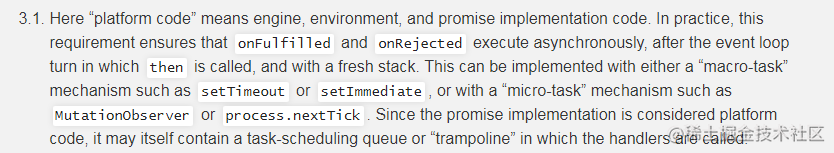3、问题1-7，我们可以采取统一的觉得方案，定义一个函数来判断和处理这一系列的情况，官方给出了一个叫做resolvePromise的函数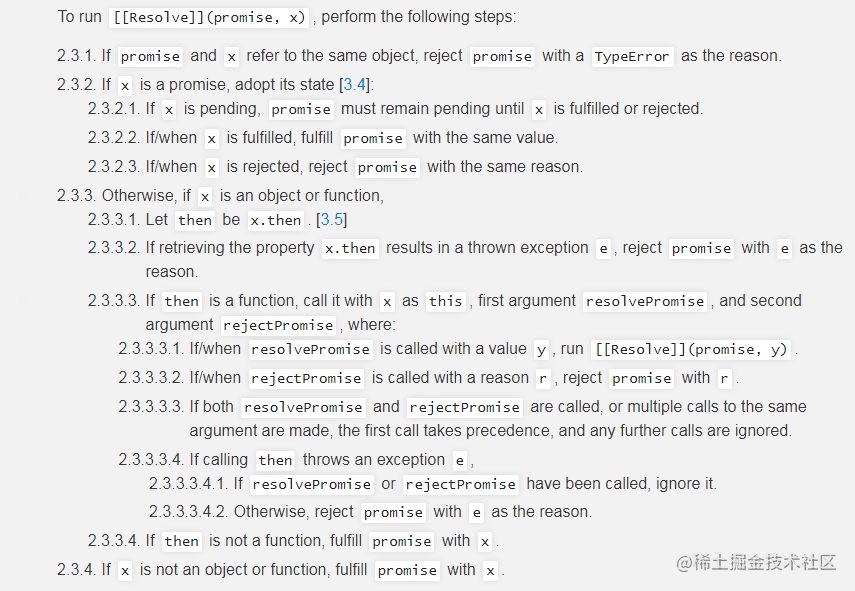``````Promise.prototype.then = function (onFulfilled, onRjected) {
//成功和失败默认不传给一个函数，解决了问题8
onFulfilled = typeof onFulfilled === 'function' ? onFulfilled : function (value) {
return value;
}
onRjected = typeof onRjected === 'function' ? onRjected : function (err) {
throw err;
}
let _this = this;
let promise2; //返回的promise
if (_this.status === 'resolved') {
promise2 = new Promise(function (resolve, reject) {
// 当成功或者失败执行时有异常那么返回的promise应该处于失败状态
setTimeout(function () {// 根据规范让那俩家伙异步执行
try {
let x = onFulfilled(_this.value);//这里解释过了
// 写一个方法统一处理问题1-7
resolvePromise(promise2, x, resolve, reject);
} catch (e) {
reject(e);
}
})
})
}
if (_this.status === 'rejected') {
promise2 = new Promise(function (resolve, reject) {
setTimeout(function () {
try {
let x = onRjected(_this.reason);
resolvePromise(promise2, x, resolve, reject);
} catch (e) {
reject(e);
}
})
})
}
if (_this.status === 'pending') {
promise2 = new Promise(function (resolve, reject) {
_this.onResolvedCallbacks.push(function () {
setTimeout(function () {
try {
let x = onFulfilled(_this.value);
resolvePromise(promise2, x, resolve, reject);
} catch (e) {
reject(e)
}
})
});
_this.onRejectedCallbacks.push(function () {
setTimeout(function () {
try {
let x = onRjected(_this.reason);
resolvePromise(promise2, x, resolve, reject);
} catch (e) {
reject(e);
}
})
});
})
}
return promise2;
}
``````

``````function resolvePromise(promise2, x, resolve, reject) {
// 接受四个参数，新Promise、返回值，成功和失败的回调
// 有可能这里返回的x是别人的promise
// 尽可能允许其他乱写
if (promise2 === x) { //这里应该报一个类型错误，来解决问题4
return reject(new TypeError('循环引用了'))
}
// 看x是不是一个promise,promise应该是一个对象
let called; // 表示是否调用过成功或者失败，用来解决问题7
//下面判断上一次then返回的是普通值还是函数，来解决问题1、2
if (x !== null && (typeof x === 'object' || typeof x === 'function')) {
// 可能是promise {},看这个对象中是否有then方法，如果有then我就认为他是promise了
try {
let then = x.then;// 保存一下x的then方法
if (typeof then === 'function') {
// 成功
//这里的y也是官方规范，如果还是promise，可以当下一次的x使用
//用call方法修改指针为x，否则this指向window
then.call(x, function (y) {
if (called) return //如果调用过就return掉
called = true
// y可能还是一个promise，在去解析直到返回的是一个普通值
resolvePromise(promise2, y, resolve, reject)//递归调用，解决了问题6
}, function (err) { //失败
if (called) return
called = true
reject(err);
})
} else {
resolve(x)
}
} catch (e) {
if (called) return
called = true;
reject(e);
}
} else { // 说明是一个普通值1
resolve(x); // 表示成功了
}
}
``````
• 测试一下

PromiseA+提供了测试库promises-aplus-tests，github上明确讲解了使用方法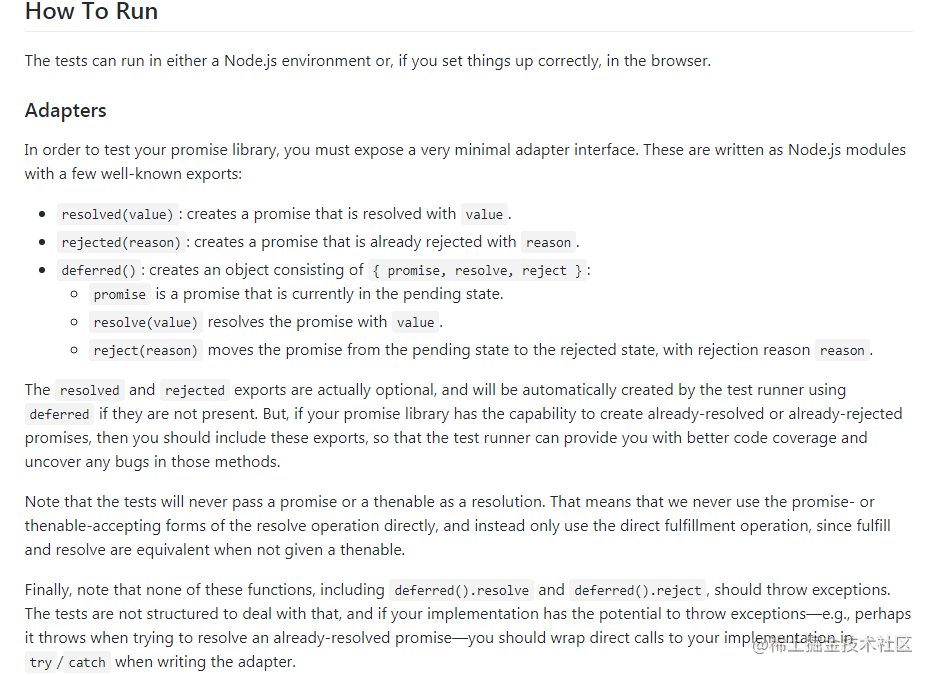公开一个适配器接口：

``````Promise.deferred = function () {
let dfd = {};
dfd.promise = new Promise(function (resolve, reject) {
dfd.resolve = resolve;
dfd.reject = reject;
});
return dfd
}
``````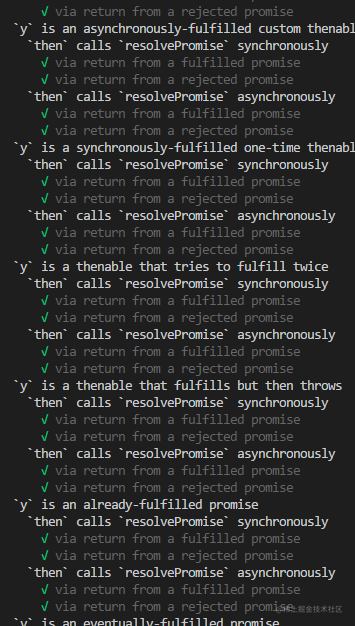``````872 passing (18s)
``````

• 其他方法

``````    // 捕获错误的方法，在原型上有catch方法，返回一个没有resolve的then结果即可
Promise.prototype.catch = function (callback) {
return this.then(null, callback)
}
// 解析全部方法，接收一个Promise数组promises,返回新的Promise，遍历数组，都完成再resolve
Promise.all = function (promises) {
//promises是一个promise的数组
return new Promise(function (resolve, reject) {
let arr = []; //arr是最终返回值的结果
let i = 0; // 表示成功了多少次
function processData(index, y) {
arr[index] = y;
if (++i === promises.length) {
resolve(arr);
}
}
for (let i = 0; i < promises.length; i++) {
promises[i].then(function (y) {
processData(i, y)
}, reject)
}
})
}
// 只要有一个promise成功了 就算成功。如果第一个失败了就失败了
Promise.race = function (promises) {
return new Promise(function (resolve, reject) {
for (var i = 0; i < promises.length; i++) {
promises[i].then(resolve,reject)
}
})
}
// 生成一个成功的promise
Promise.resolve = function(value){
return new Promise(function(resolve,reject){
resolve(value);
})
}
// 生成一个失败的promise
Promise.reject = function(reason){
return new Promise(function(resolve,reject){
reject(reason);
})
}
``````欢迎加我的个人微信深入交流